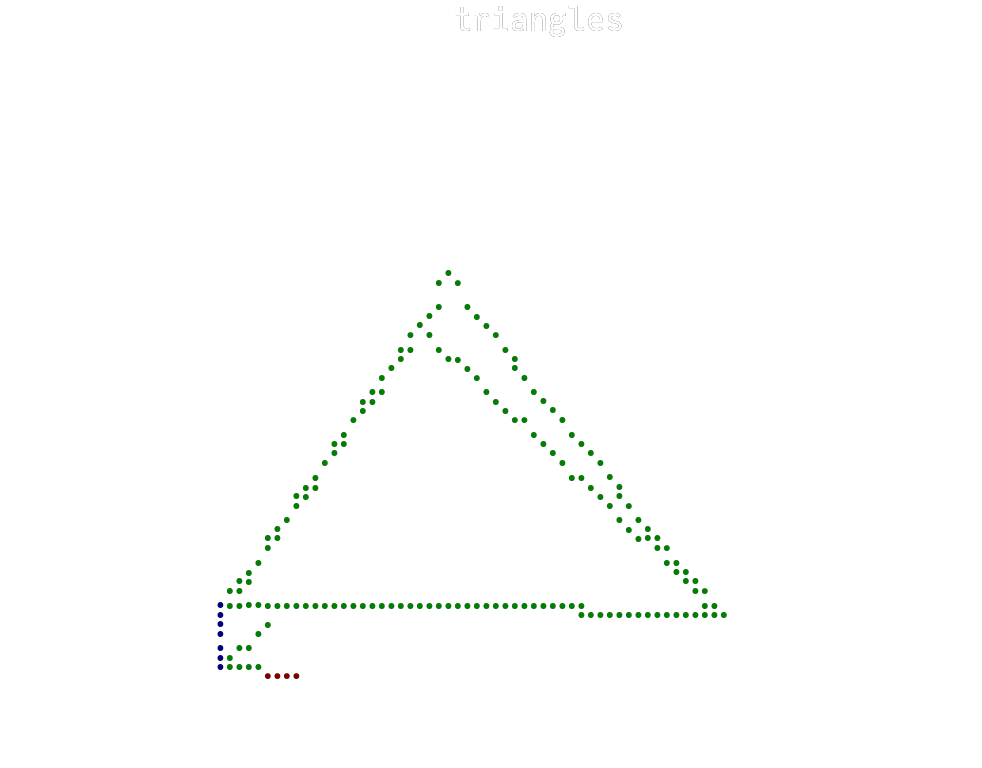# Mesh3dusing Plots
unicodeplots()

Allows to plot arbitrary 3d meshes. If only x,y,z are given the mesh is generated automatically. You can also specify the connections using the connections keyword. The connections can be specified in two ways: Either as a tuple of vectors where each vector contains the 0-based indices of one point of a triangle, such that elements at the same position of these vectors form a triangle. Or as a vector of NTuple{3,Ints} where each element contains the 1-based indices of the three points of a triangle.

x = [0, 1, 2, 0]
y = [0, 0, 1, 2]
z = [0, 2, 0, 1]
i = [0, 0, 0, 1]
j = [1, 2, 3, 2]
k = [2, 3, 1, 3]
mesh3d(x, y, z; connections = (i, j, k), title = "triangles", xlabel = "x", ylabel = "y", zlabel = "z", legend = :none, margin = 2 * Plots.mm)This page was generated using DemoCards.jl and Literate.jl.